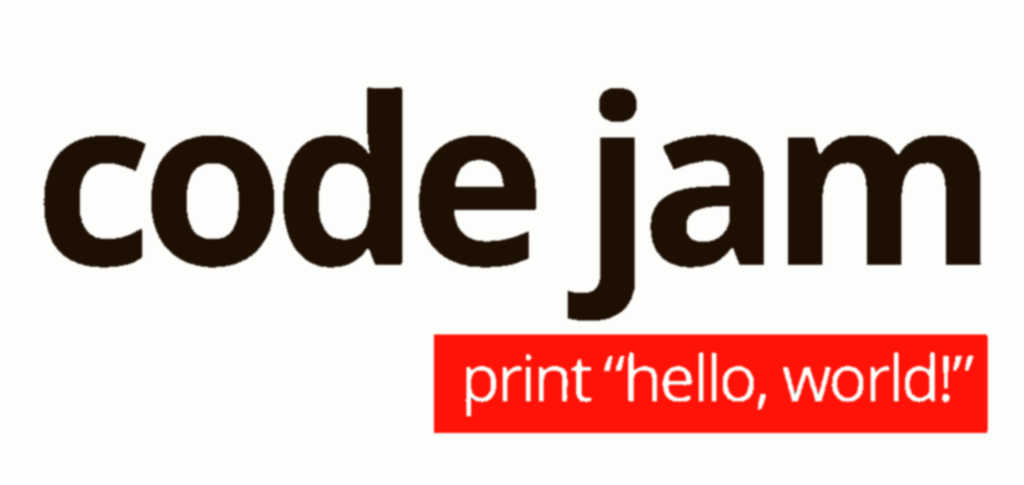Broken Clock2021-04-28T21:42:04+02:00
Code Jam gcj2021

Round 1B’s problem A, “Broken Clock”, gives us a clock’s position and asks us for the time.

Catch number 1: it doesn’t tell us which hand is which. Countermeasure number 1: there are only 3 hands, that’s 6 permutations. We can try them all and keep the consistent ones only.

Catch number 2: the clock has no markings, so all hand positions are given with an arbitrary offset. Countermeasure 2: that offset is eliminated by pairwise subtraction.

So let’s get the math out. The clock positions are given in nanoseconds, $$360×12×10^{10}$$ per revolution. For obvious reasons, I’ll call that constant $$2\pi$$. For a time $$t$$ in nanoseconds and hand positions $$h$$, $$m$$ and $$s$$, we’d have:

$\left\{ \begin{array}{cclc} h & = & \theta + t & [\bmod 2\pi] \\ m & = & \theta + 12t & [\bmod 2\pi] \\ s & = & \theta + 720t & [\bmod 2\pi] \end{array} \right.$

Subtracting pairwise,

$\left\{ \begin{array}{cclc} m - h & = & 11t & [\bmod 2\pi] \\ s - m & = & 708t & [\bmod 2\pi] \\ s - h & = & 719t & [\bmod 2\pi] \end{array} \right.$

Three equations, four unknowns. But $$t$$’s range is bounded, so there’s probably a way to get a MIP solver on the task. Luckily for us, that won’t be needed.

The variables are all integers.1 So we’re really looking at a modular inverse. The 708 may be a bit of a composite, but 11 and 719 are bona-fide primes, we can invert them without a second thought using any conventional means: product enumeration, exponentiation, WolframAlpha2, extended Euclid/Bézout.3

I suppose 128-bit integers would be ok, but they’re a bit of a pain to get working in Haskell. Especially when we’ve got BigInt baked right in.

twoPi,eleven_ :: Integer
twoPi = 360 * 12 * 10^10
eleven_ = 15709090909091

Now given $$h$$, $$m$$ and $$s$$, we can obtain $$t$$ directy from just a single difference (the hours-minutes one), there is always one that fits. Then we can trivially verify the seconds hand is indeed in the correct position relative to the others for that time. Any equation involving $$s$$ and $$t$$ will do, though testing both for acute decision fatigue also works.

verify :: [Integer] -> Maybe Integer
verify [h,m,s] = do
let t = (m - h) * eleven_ mod twoPi
guard $((720-1) * t - (s - h)) mod twoPi == 0 guard$ ((720-12) * t - (s - m)) mod twoPi == 0
pure t

Solving from $$A$$, $$B$$, $$C$$ is a simple matter of trying the permutations until a fit is found. The judge accepts any solution if there are multiple.4

solve :: Integer -> Integer -> Integer -> (Integer,Integer,Integer,Integer)
solve a b c = (h,m,s,n) where
(t',n) = t divMod (10^9)
(t'',s) = t' divMod 60
(h,m) = t'' divMod 60
t = head $mapMaybe verify$ permutations [a,b,c]

Here’s a fairly standard CodeJam wrapper for completeness.

main :: IO ()
main = do
forM_ [1..t] $\i -> do [a,b,c] <- map read . words <$> getLine
let (h,m,s,n) = solve a b c
putStrLn \$ "Case #" ++ show i ++ ": " ++ unwords (map show [h,m,s,n])

This concludes this puzzle’s solution. As usual, the full code is on GitHub. See you soon for the round’s other problems!

1. Including $$2\pi$$. Yeah, I know.↩︎

2. Which happened to be my pick.↩︎

3. One of those is a joke, BTW. Not that it wouldn’t work, but…↩︎

4. I had to re-read the statement multiple times until I noticed it, though.↩︎